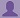SHARE
HELP

To start with, Sr2Jr’s first step is to reduce the expenses related to education. To achieve this goal Sr2Jr organized the textbook’s question and answers. Sr2Jr is community based and need your support to fill the question and answers. The question and answers posted will be available free of cost to all.# Computer Networking : A Top-down Approach

Authors:
James F. Kurose, Keith W. Ross
Exercise:
Review
Chapter:
Computer Networks And The Internet
Edition:
6
ISBN:
9780132856201
Question:
19
 Previous Next

###Question

Suppose Host A wants to send a large file to Host B. The path from Host A to Host B has three links, of rates R1 = 500 kbps, R2 = 2 Mbps, and R3 = 1 Mbps.

a. Assuming no other traffic in the network, what is the throughput for the file transfer?

b. Suppose the file is 4 million bytes. Dividing the file size by the throughput,roughly how long will it take to transfer the file to Host B?

c. Repeat (a) and (b), but now with R2 reduced to 100 kbps.

###Answer

a)

Consider givend data:R1 = 500 kbps, R2 = 2 Mbps, and R3 = 1 Mbps

The throughput for the file transfer=min{R1,R2,R3}

=min{500 kbps, 2 Mbps, 1 Mbps}

=500 kbps

So, the throughput for the file transfer=500 kbps

b)

Consider given data:

The file size= 4 million bytes

Convert million bytes to bits

=32000000 bits.

From (a), Throughput for the file transfer=500 Kbps

=500000 bps

Dividing the file size by the throughput,roughly how long will it take to transfer the file to Host B:

=file size/hroughput for the file transfer

=32000000 bits/500000 bps

=64 seconds

c)

Consider the given data:

Repeat (a) and (b), but now with R2 reduced to 100 kbps.

That means R2=100 kbps , R1 = 500 kbps,  and R3 = 1 Mbps

The throughput for the file transfer=min{R1,R2,R3}

=min{500 kbps, 100 kbps, 1 Mbps}

=100 kbps

So, the throughput for the file transfer=100 kbps

Dividing the file size by the throughput,roughly how long will it take to transfer the file to Host B:

=file size/hroughput for the file transfer

=32000000 bits/100000 bps

=320 seconds

### Discussions

Post the discussion to improve the above solution.